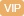| APP | 校园号 | 客服 客服热线：400-863-9889

400-863-9889100-23=230+460=76-47=3000+200=420-80=
 5 9
+
 2 9
=
1-
 1 7
=
248-108≈352+295≈308+107≈
【考点】整数的加法和减法数的估算分数的加法和减法【专题】计算题．
【分析】根据数加减乘除法的计方进行答10-25-75运用减法的行简算，1000÷1258运除法的性质进行简算，999×+运用乘法的配律进简即可．
【解答】解：
 100÷8=25 5+0.25=0.75 10-.36=9.4 2550=250 16+840=000 350-180=0 -2.5=3.46 720090=0 3504=140 10025-7=0 100125÷8=1 9×9+9=90000
【点评】此题考了数加减乘除的口算能力灵活运用运算定进行算即可．

0/0